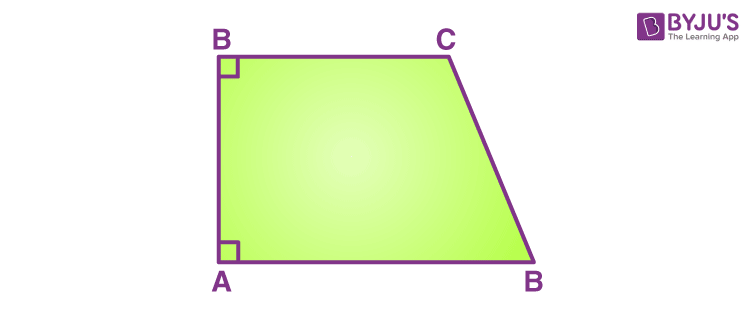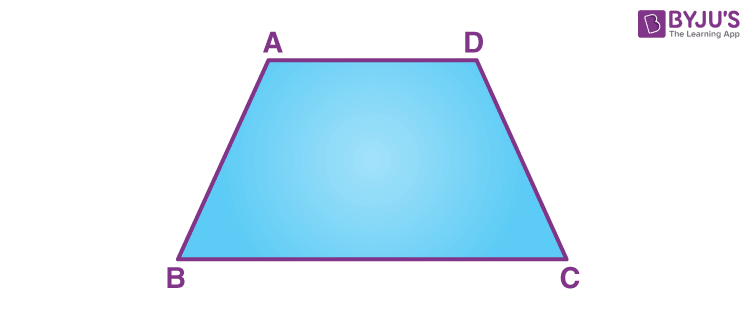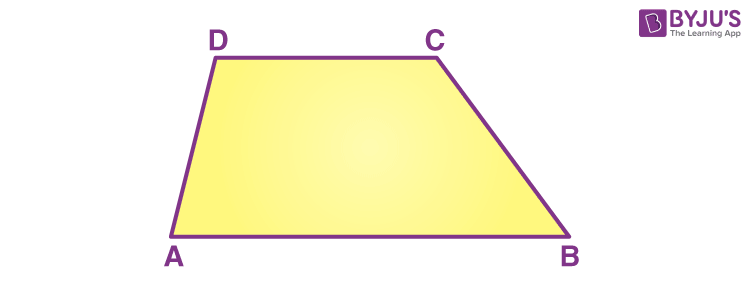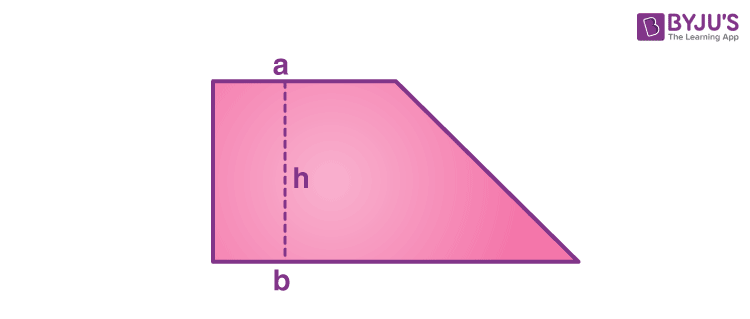# Trapezoids

Trapezoids are quadrilaterals that have two parallel sides and two non-parallel sides. It is also called a Trapezium. A trapezoid is a four-sided closed shape or figure which cover some area and also has its perimeter. It is a 2D figure and not 3D figure. The sides which are parallel to each other are termed the bases of the trapezoid. The non-parallel sides are known as legs or lateral sides. The distance between the parallel sides is known as the altitude. The area of trapezium along with its types, properties and other trapezoids-related formulas are provided here in this article.

## What is a Trapezoid?

A trapezoid is a polygon that has only one pair of parallel sides. These parallel sides are also called parallel bases of trapezoid. The other two sides of trapezoids are non-parallel and called legs of trapezoids.

There is some disagreement over the definition of trapezoids. One school of mathematics considers that a trapezoid can have one and only one pair of parallel sides, while the other argues that there can be more than one pair of parallel sides in a trapezoid. If we consider the second definition, a parallelogram is also a trapezoid according to that. But the first definition does not consider a parallelogram to be a trapezoid. Since we have already mentioned it as one of the types of quadrilaterals.

## Types of Trapezoids

Trapezoids can be broadly classified into three groups-

• Right Trapezoids
• Isosceles Trapezoids
• Scalene Trapezoids

### Right Trapezoids

A Right trapezoid is a trapezoid that has a pair of right angles, adjacent to each other. It is used while evaluating the area under the curve, under that trapezoidal rule.### Isosceles Trapezoids

If the non-parallel sides, or we can say, the legs of a trapezoid are equal in length, it is known as an Isosceles trapezoid.### Scalene Trapezoids

When neither the sides nor the angles of a trapezium are equal, we call it a Scalene trapezoid.## Shape of Trapezoids

A trapezoid is a four-sided shape which has one pair of sides as parallel. It is basically a two-dimensional shape or figure similar to a square, rectangle, parallelogram. Hence, this shape also has its perimeter and area as other shapes do. Let us see the formula for its area and perimeter. Students can learn the comparison between parallelogram, trapezium and kite from here.

## Trapezoids Formulas

The two major formulas related to trapezoids are:

• Area of Trapezoid
• Perimeter of Trapezoid

### Area of Trapezoid

The area of a trapezoid can be calculated by taking the average of the two bases and multiplying it with the altitude. The area formula for trapezoids is given by-Area = 1/2 (a+b) h

### Perimeter of Trapezoid

The perimeter of a trapezoid is the sum of all its sides. Therefore, for a trapezoid with sides a, b, c and d, the formula of the perimeter can be written as-

Perimeter= a + b + c + d

### Midsegment and height

The alternate name for midsegment is the median of a trapezoid. It is the part that connects the midpoints of the limbs. The median is parallel to the bases. The length that is called “m” is the same as the mean of the base lengths a and b of the trapezoid.

$$\begin{array}{l}m = \frac{a + b}{2}.\end{array}$$

The height or alternatively called the altitude is the perpendicular distance connecting the bases. If the bases possess varied lengths, then the altitude of the trapezoid h can be found by the lengths of 4 sides by the formula

$$\begin{array}{l}h= \frac{\sqrt{(-a+b+c+d)(a-b+c+d)(a-b+c-d)(a-b-c+d)}}{2|b-a|}\end{array}$$

Here c and d refer to the lengths of the limbs.

### Diagonals of Trapezoid

The diagonal lengths can be found by the formulae

$$\begin{array}{l}p= \sqrt{\frac{ab^2-a^2b-ac^2+bd^2}{b-a}},\\ q={\sqrt {\frac {ab^{2}-a^{2}b-ad^{2}+bc^{2}}{b-a}}}}\end{array}$$

Here a is the base that is shorter and b is the base that is longer, c & d are limbs of the trapezoid.

## Properties of Trapezoids

There are certain properties of trapezoids that identify them as trapezoids:

• The base angles and the diagonals of an isosceles trapezoid are equal.
• If you draw a median on a trapezoid, it will be parallel to the bases and its length will be the average of the length of the bases.
• The intersection point of the diagonals is collinear to the midpoints of the two opposite sides.
• If there is a trapezoid with sides a, b, c and d and diagonals p and q, the following equation will be true: p2+q2= c2+d2+2ab
• The center of the area that is present through the line segment connecting the midpoints of the sides that are parallel, at a perpendicular distance x from the side that is larger named b is given by:
$$\begin{array}{l}x = \frac{h}{3} \left( \frac{2a+b}{a+b}\right)\end{array}$$
• $$\begin{array}{l}\text{It divides the segment in the ratio }{\frac {a+2b}{2a+b}}}\end{array}$$
• If the bisectors of the angles A and B bisect at P and the bisectors of the angles C and D bisect at Q, then
$$\begin{array}{l}PQ=\frac{|AD+BC-AB-CD|}{2}\end{array}$$

## Solved Examples on Trapezoids

Example 1: Find the area of a trapezoid with bases of 3 meters and 5 meters and a height of 4 meters.

Solution:

We know that the area of a Trapezoid is 1/2 (a+b) × h
Area = (3+5)/2 × 4 (m2)
Area = 16 m2
Example 2: A trapezoid has four sides measuring 3m, 5m, 7m and 4m. Find its perimeter.

Solution:

We know that Perimeter is given by Sum of all the sides.

Therefore, Perimeter = 3+5+7+4

= 19 m

Example 3: Is a parallelogram also a trapezoid?

Solution: No, a trapezoid is not a parallelogram.

As per definition:

Parallelogram has two pairs of parallel sides, while Trapezoid has Exactly two parallel sides.

Example 4: What is the area of a trapezium with the parallel side lengths 10 and 12 cm and the distance between them is 4 centimeters?

Answer: By the formula we know that;

Area of trapezoid = 1/2 × (sum of the parallel sides) × (Distance between the parallel sides)

= 1/2 × (10 + 12) × 4

= 1/2 × 22 × 4

= 11 × 4

= 44 cm2

Example 5: Given the area of a trapezium to be 440 square centimeters. The parallel side lengths are 30 and 14 centimeters. What is the distance between them?

Answer: Assume the distance between them to be h cm.

Since,

Area of trapezoid = 1/2 × (sum of the parallel sides) × (Distance between the parallel sides)

440 = 1/2 × (30 + 14) × h

h = (440 × 2)/44 cm

h = 10 × 2  cm

h = 20 cm

Example 6: The area of a trapezium is given to be 480 square meters. The height is 15 meters and the value of one of the sides is 20 meters. What is the value of the other side?

Solution: By the formula, we know;

Area of trapezoid = 1/2 × (sum of the parallel sides) × (Distance between the parallel sides)

480 = 1/2 × (side 1 + side 2) × 15

480 = 1/2 × (20 + side 2) × 15

20 + side 2 = (480 × 2)/15

20 + side 2 = 32 × 2

20 + side 2 = 64

side 2 = 44 cm

## Frequently Asked Questions on Trapezoids

Q1

### What are Trapezoids?

Trapezoids are the 4-sided polygons which have two parallel sides and two-non parallel sides. It is also called a Trapezium, sometimes.

Q2

### What is the area of a trapezoid?

The area of a trapezoid can be determined by taking the average of the two parallel bases and multiplying it with the altitude or distance between the two parallel sides.

Q3

Yes, a trapezoid is a quadrilateral who has its two sides parallel and the other two sides are non-parallel.

Q4

### What are the three attributes of trapezoids?

The base angles and the diagonals of an isosceles trapezoid are equal.
The intersection point of the diagonals is collinear to the midpoints of the two opposite sides.
Opposite sides of an isosceles trapezoid are of the same length or congruent to each other.